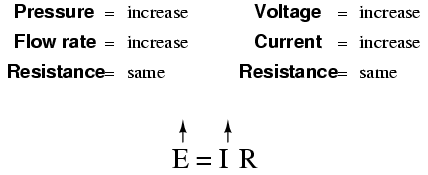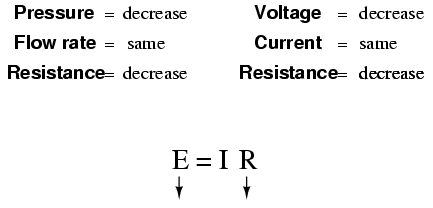## What is the analogy of Ohm's Law?

The water-and-pipe analogy. If we have a water pump that exerts pressure (voltage) to push water around a "circuit" (current) through a restriction (resistance), we can model how the three variables interrelate.

# An Analogy for Ohm’s Law

## Chapter 2 - Ohm's Law

Ohm’s Law also makes intuitive sense if you apply it to the water-and-pipe analogy. If we have a water pump that exerts pressure (voltage) to push water around a “circuit” (current) through a restriction (resistance), we can model how the three variables interrelate.

If the resistance to water flow stays the same and the pump pressure increases, the flow rate must also increase.If the pressure stays the same and the resistance increases (making it more difficult for the water to flow), then the flow rate must decrease:If the flow rate were to stay the same while the resistance to flow decreased, the required pressure from the pump would necessarily decrease:As odd as it may seem, the actual mathematical relationship between pressure, flow, and resistance is actually more complex for fluids like water than it is for electric charge. If you pursue further studies in physics, you will discover this for yourself. Thankfully for the electronics student, the mathematics of Ohm’s Law is very straightforward and simple.

REVIEW:

• With resistance steady, current follows voltage (an increase in voltage means an increase in current, and vice versa).
• With voltage steady, changes in current and resistance are opposite (an increase in current means a decrease in resistance, and vice versa).
• With current steady, voltage follows resistance (an increase in resistance means an increase in voltage).

RELATED WORKSHEETS:

Try out our Ohm’s Law Calculator in our Tools section.

• Share
Published under the terms and conditions of the Design Science License